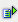The function avg (range) calculates the average of the values within a range.

Syntax: =avg(range)Example

Returns the average in the defined range.

Cell A4 = avg(A1:A3)

 R / C A 1 1 2 3 3 5 4 3

The average of the cells A1 to A3 is 3